Examples

Chapter 3 Class 10 Pair of Linear Equations in Two Variables
Serial order wise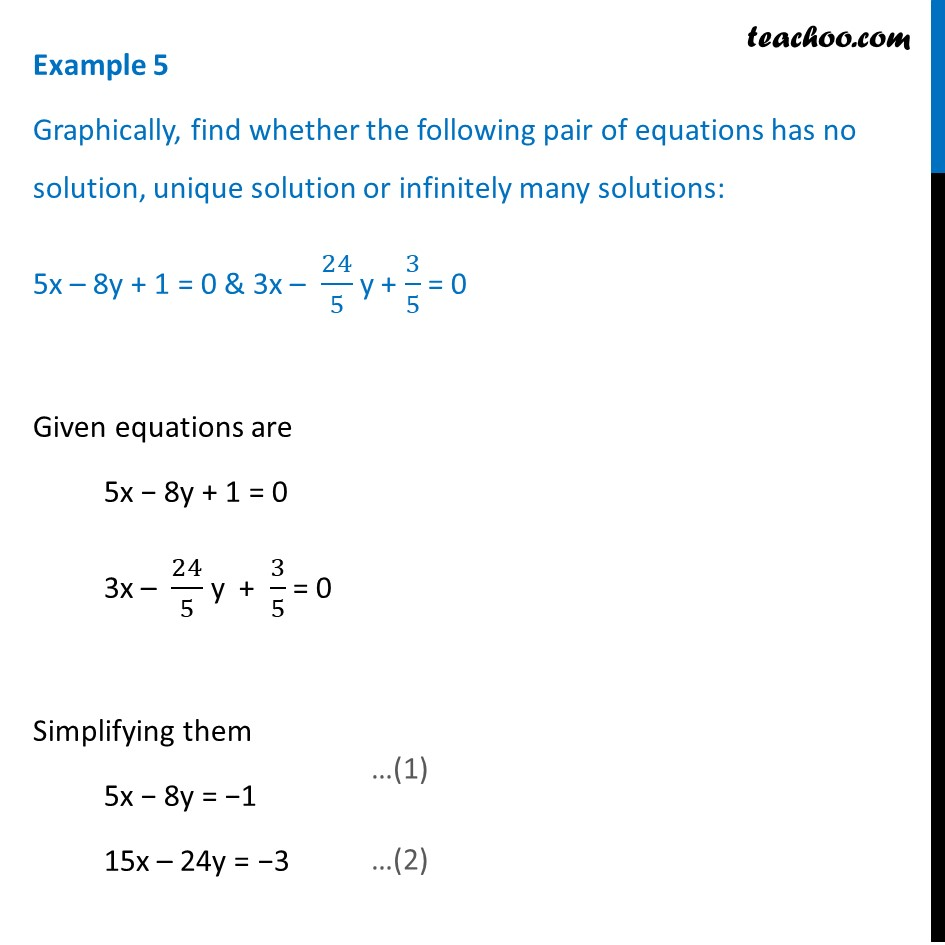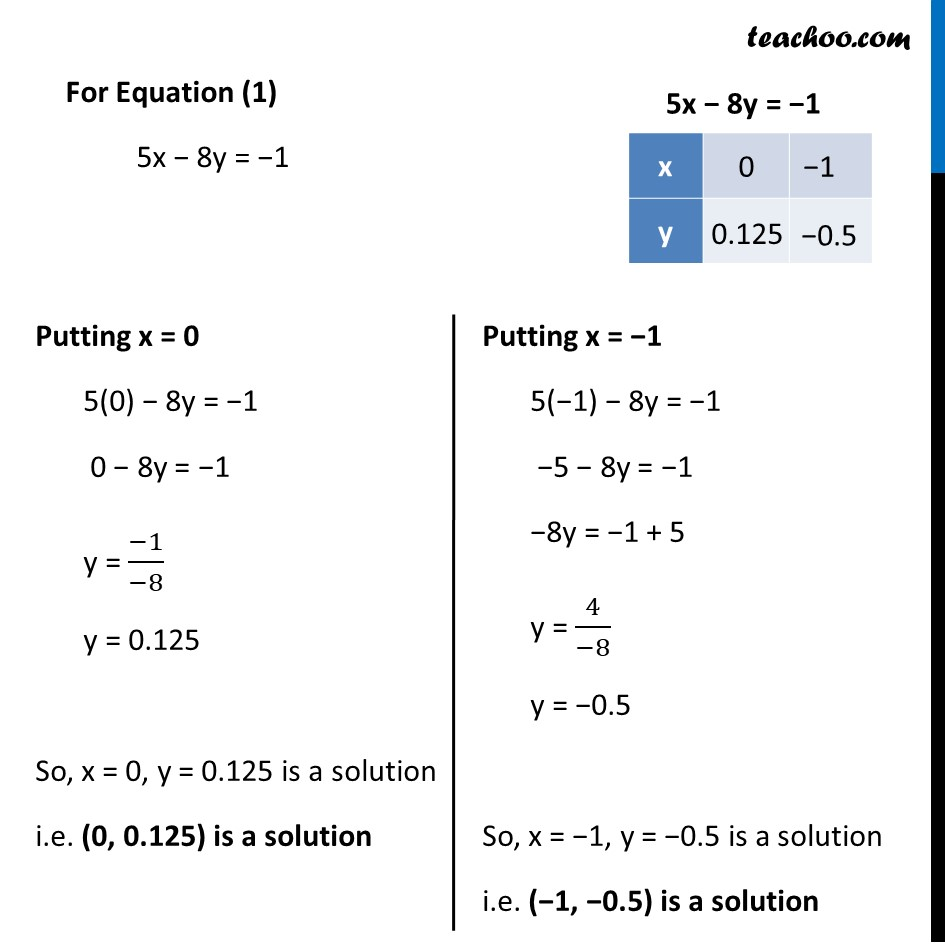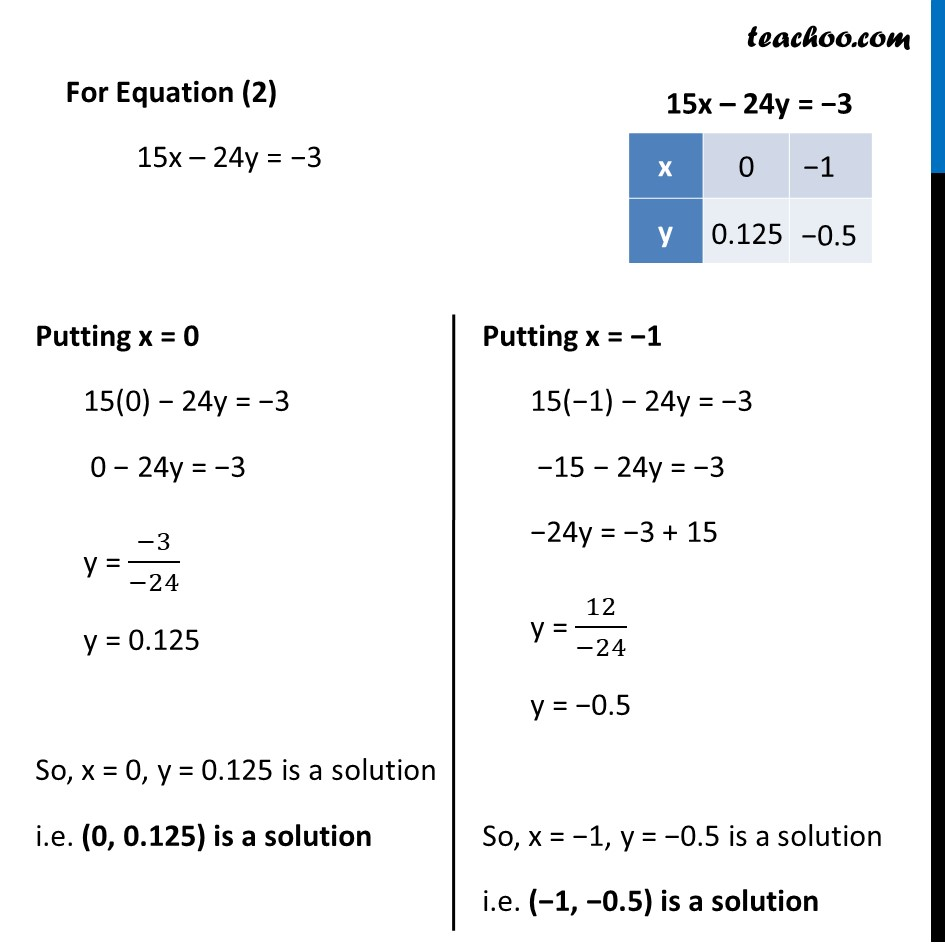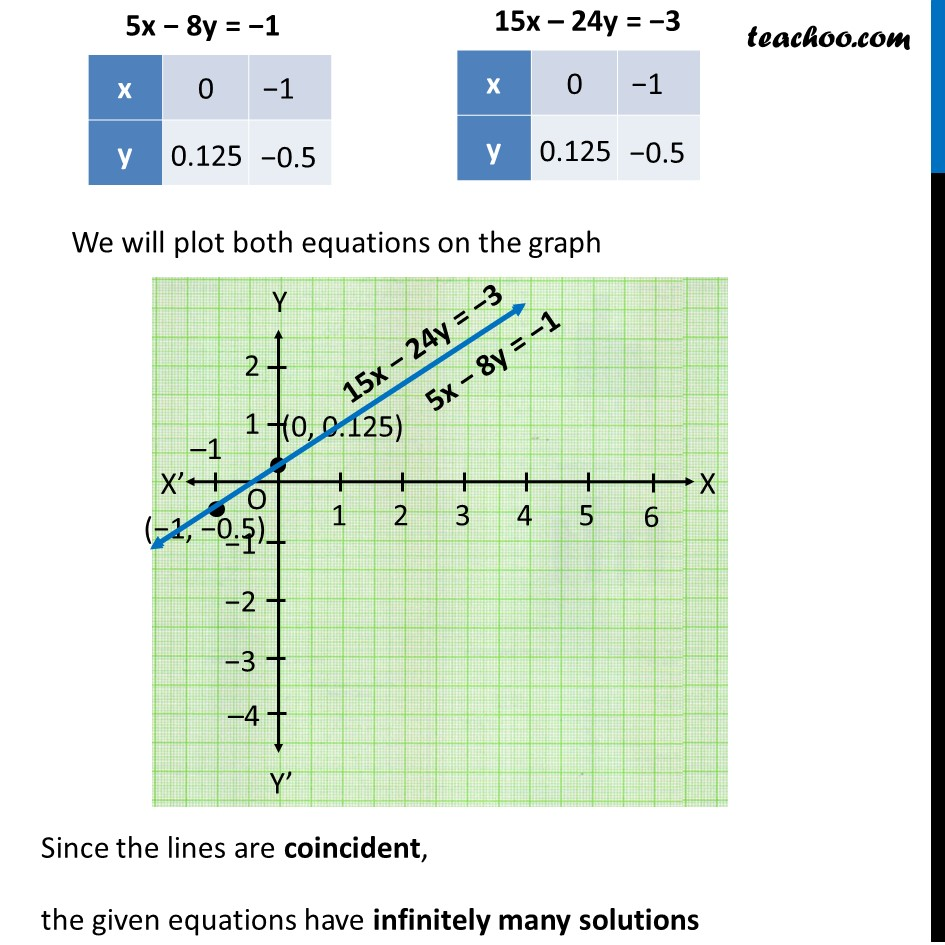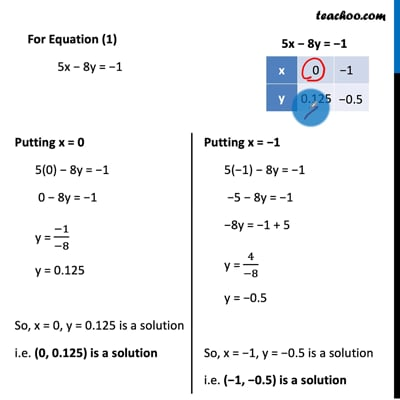This video is only available for Teachoo black users

Solve all your doubts with Teachoo Black (new monthly pack available now!)

### Transcript

Example 5 Graphically, find whether the following pair of equations has no solution, unique solution or infinitely many solutions: 5x – 8y + 1 = 0 & 3x – 24/5 y + 3/5 = 0 Given equations are 5x − 8y + 1 = 0 3x – 24/5 y + 3/5 = 0 Simplifying them 5x − 8y = −1 15x – 24y = −3 For Equation (1) 5x − 8y = −1 Putting x = 0 5(0) − 8y = −1 0 − 8y = −1 y = (−1)/(−8) y = 0.125 So, x = 0, y = 0.125 is a solution i.e. (0, 0.125) is a solution Putting x = −1 5(−1) − 8y = −1 −5 − 8y = −1 −8y = −1 + 5 y = 4/(−8) y = −0.5 So, x = −1, y = −0.5 is a solution i.e. (−1, −0.5) is a solution For Equation (2) 15x – 24y = −3 Putting x = 0 15(0) − 24y = −3 0 − 24y = −3 y = (−3)/(−24) y = 0.125 So, x = 0, y = 0.125 is a solution i.e. (0, 0.125) is a solution Putting x = −1 15(−1) − 24y = −3 −15 − 24y = −3 −24y = −3 + 15 y = 12/(−24) y = −0.5 So, x = −1, y = −0.5 is a solution i.e. (−1, −0.5) is a solution We will plot both equations on the graph Since the lines are coincident, the given equations have infinitely many solutions